# More Examples of Continuous-Time Convolution, Properties of Convolution

The Step Response is the response of an LTI system to a unit step function. In other words, the input to the system is simply the unit step function: x(t) = u(t).

This is equivalent to simply integrating the input from the infinite past up to time t.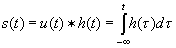Example 1

Superposition (or Divide-and-Conquer):

We can directly apply superposition to find the output of LTI systems if x(t) can be expressed as a linear combination of basis functions Φk(t).

The Φk(t) are some convenient set of functions, for example unit impulses, unit step functions, or complex exponentials.

 Example 2 If an input is written as: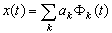using superposition, we can write its output as: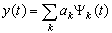where

Ψk(t) =  Sk(t)]  =  h(t) * Φk(t) =  Φk(t) *  h(t)

Again, using superposition, we can write: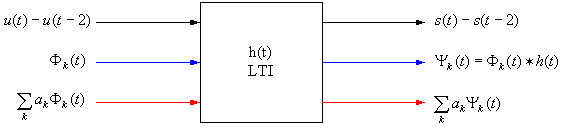Example 3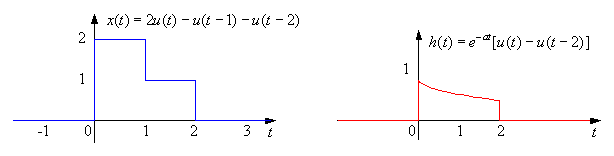Find the output of the system where

x(t) = 2u(t) - u(t - 1) - u(t - 2) and
h(t) = e-at [ u(t) - u(t - 2) ].

# Properties of Convolution

Convolution is commutative, associative, and distributive. Keeping this in mind may simplify some convolutions for you.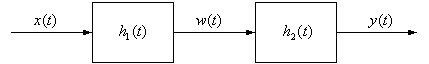w(t) = x(t) * h1(t),    y(t) = w(t) * h2(t)

= [ x(t) * h1(t) ] * h2(t)

= x(t) * [h1(t) * h2(t)], by associativity of convolution

Therefore the impulse response h(t) for this overall system is h1(t) * h2(t).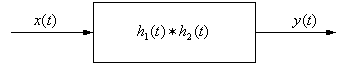We can change the order in which the convolutions are performed due to commutativity. For a cascade of M systems there are M! possible system orderings.

### Parallel interconnection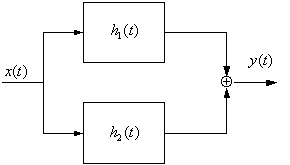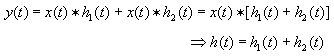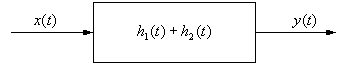Parallel systems is a large area of research today.